# Analog Electronics - 5

## 10 Questions MCQ Test GATE ECE (Electronics) 2023 Mock Test Series | Analog Electronics - 5

Description
Attempt Analog Electronics - 5 | 10 questions in 30 minutes | Mock test for Electronics and Communication Engineering (ECE) preparation | Free important questions MCQ to study GATE ECE (Electronics) 2023 Mock Test Series for Electronics and Communication Engineering (ECE) Exam | Download free PDF with solutions
QUESTION: 1

### The lower 3-dB frequency of the amplifier circuit shown below is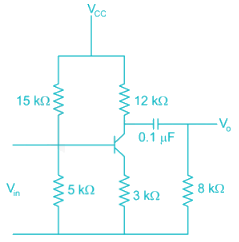Solution:

The lower 3 dB frequency ω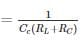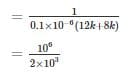*Answer can only contain numeric values
QUESTION: 2

### The efficiency of a class B amplifier with a supply voltage of 24V and peak output voltage Vo = 22V is _______%

Solution:

For a class B amplifier with VCC = 24 V and VO = 22 V efficiency η (in %) is given by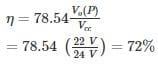QUESTION: 3

### In class AB amplifiers, the current flows through the active device for

Solution:

For class A the angle of excursion is 3600

For class B the angle of excursion is 1800

For class AB the angle of excursion is >1800 and < 3600

For class C the angle of excursion is less than 1800

*Answer can only contain numeric values
QUESTION: 4

A class – A transformer coupled, the Transistor power amplifier is Required to deliver a power output of 10 watts, the maximum power rutting of the transistor should not be less than –

Solution:

PiDC = Poac + PTr

Pooc = 10 W

η = 50% for class-A transformer coupled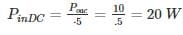QUESTION: 5

In the following transistor circuit,VBE = 0.7 V, rπ = 25 mV / IE, and β and all the capacitances are very large

The mid-band voltage gain of the amplifier is approximately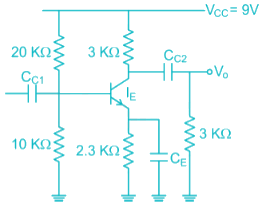Solution:

Apply ac analysis to calculate gain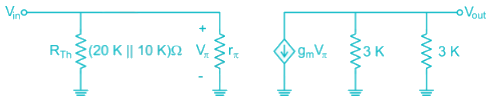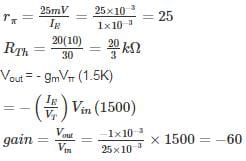∴ Option (4) is correct.

QUESTION: 6

A Transformer coupled class A amplifier has a loudspeaker of 8 Ω connected or across its secondary. The Q point of collector current is 140 mA. The Turns ratio of the transformer is 3:1. If the power delivered to the load is 0.48 W. Then the rms voltage at the primary and efficiency of the transformer is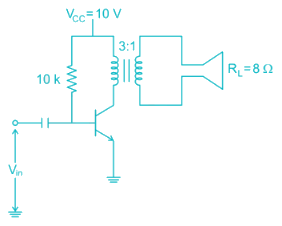Solution:

Given

PAC(secondary) = 0.48 W

ICQ = 140 mA

RL = 8 Ω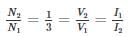PAC(sec) = PAC(primary)

PAC(primary) = 0.48 W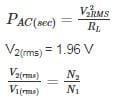V1(rms) = 1.96 × 3 = 5.88 V

output DC power

PDC = VCC ICQ

= 10(140 × 10-3)

= 1.4 W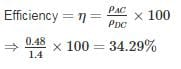*Answer can only contain numeric values
QUESTION: 7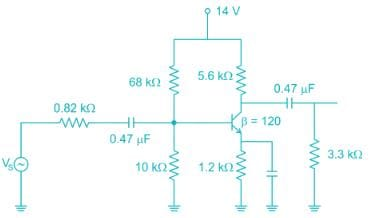The low frequency cut-off frequency due to source capacitance (0.47 uF) is ________ Hz

Take VT = 26 mV. ( assume 0.47 uF capacitance at input is source capacitance )

Solution:

The cut-off frequency due to source capacitance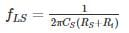Where RS = source resistance

Ri = Input impedance

Where Ri = R1 // R2// βre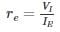In DC bias values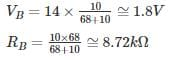Taking KVL in base-emitter path

VB - VBE - IE (1.2 Ω) = 0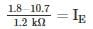IE = 0.917 mA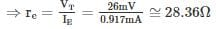∴ Ri = R1 // R2 // βve

= 10 kΩ // 68 kΩ // (120 × 28.36) Ω

≅ 2.44 k Ω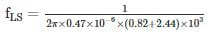= 103. 87 Hz

QUESTION: 8

For a class A transformer coupled amplifier calculate the maximum power delivered to the load when its RL = 10 Ω, n = 5 (turns ratio) and VCC = 16 V

Solution:

For class A transformer coupled amplifier

η = 50%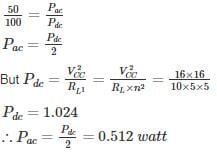QUESTION: 9

The amplifier is shown below as a voltage gain of -2.5, and input resistance of 10 kΩ and a lower 3 dB cut-off frequency of 20 Hz. Which on the following statements is TRUE when the emitter resistance RE is doubled?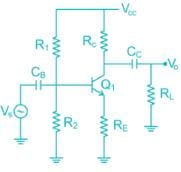Solution:

The Voltage Gain of the common emitter amplifier is equal to the ratio of the change in the input voltage to the change in the amplifiers output voltage. Then ΔVL is Vout and ΔVB s Vin. But voltage gain is also equal to the ratio of the signal resistance in the Collector to the signal resistance in the Emitter and is given as:

Voltage gain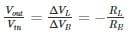∴ If RE increase then voltage gain will decrease and –ve feedback increase

*Answer can only contain numeric values
QUESTION: 10

In the circuit shown in figure find the voltage drop (in V) in 1 kΩ Resistor. Assume β = 100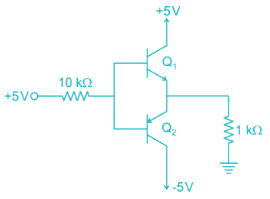Solution: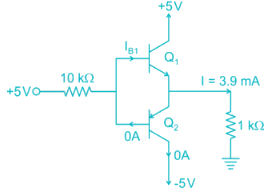Assume that Q2 in an. It fallows that current will flow from ground through the 1kΩ Resistor into the emitter of Q2. Thus the base of Q2 will be at –Ve voltage and base current will be flowing out of the through the 10 kΩ resistor and into +5V supply. This is impossible, since if the base is negative. Current in the 10 kΩ resistor will have to flow into the base. Thus we conclude that our original assumption that Q2 is ON is incorrect. It follows that Q2 will be off and Q1 will be ON.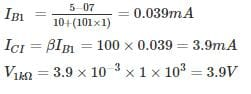Use Code STAYHOME200 and get INR 200 additional OFF Use Coupon Code